Anzeige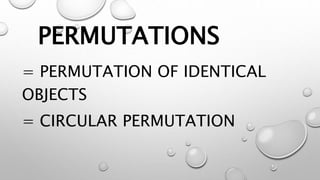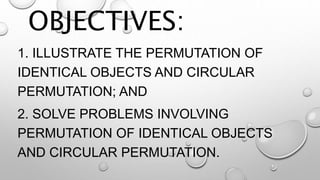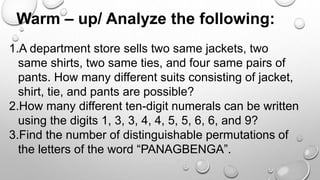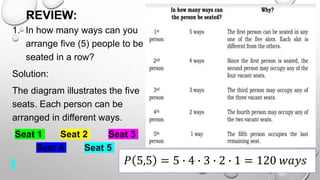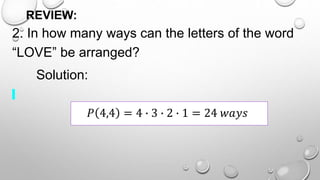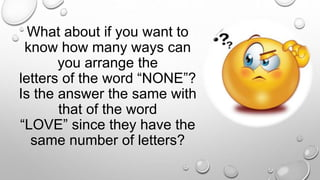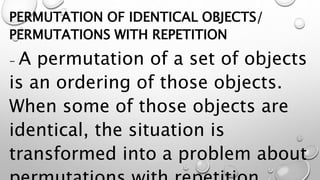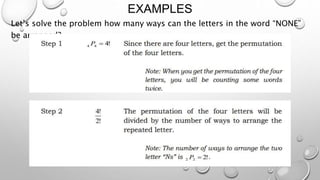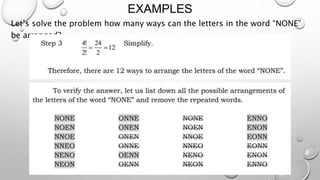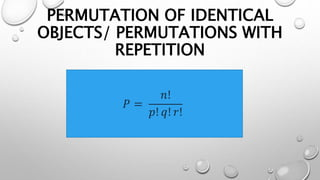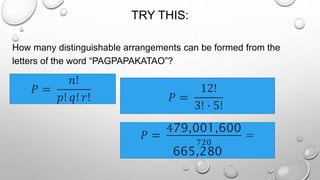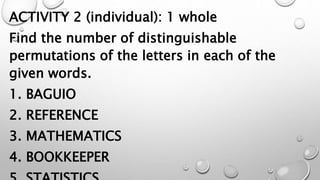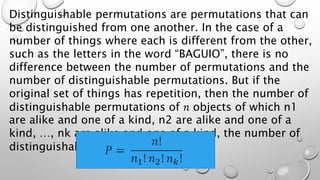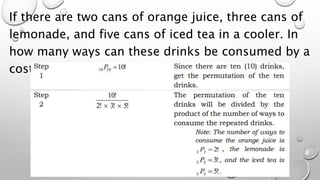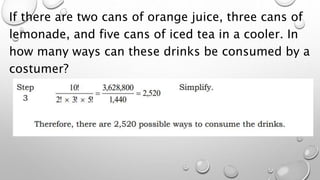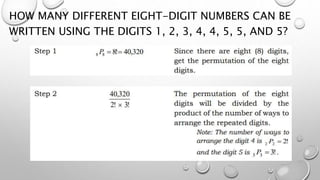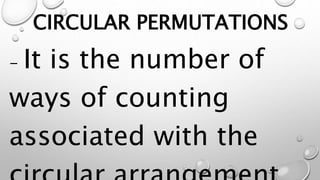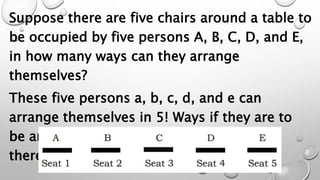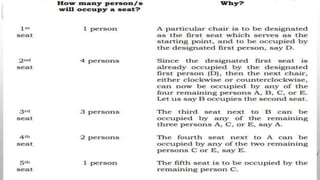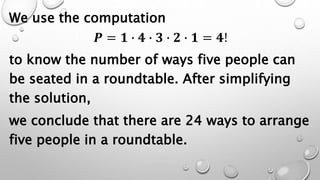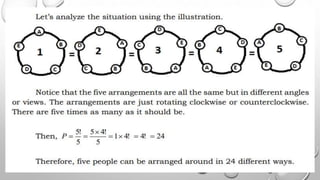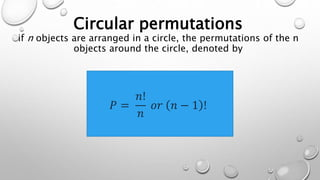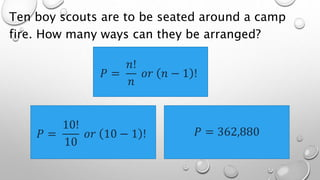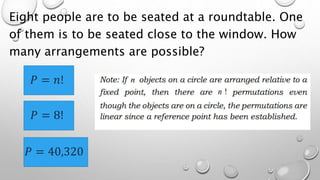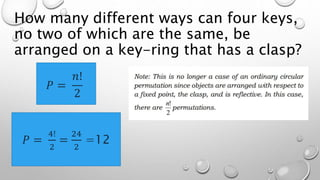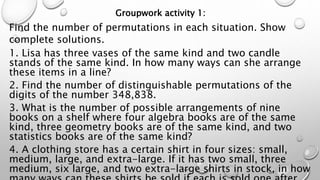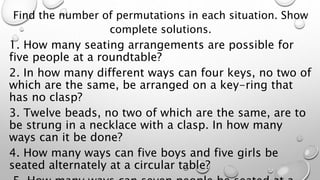1 von 28
Anzeige

### PERMUTATIONS day2.pptx

1. PERMUTATIONS = PERMUTATION OF IDENTICAL OBJECTS = CIRCULAR PERMUTATION
2. OBJECTIVES: 1. ILLUSTRATE THE PERMUTATION OF IDENTICAL OBJECTS AND CIRCULAR PERMUTATION; AND 2. SOLVE PROBLEMS INVOLVING PERMUTATION OF IDENTICAL OBJECTS AND CIRCULAR PERMUTATION.
3. Warm – up/ Analyze the following: 1.A department store sells two same jackets, two same shirts, two same ties, and four same pairs of pants. How many different suits consisting of jacket, shirt, tie, and pants are possible? 2.How many different ten-digit numerals can be written using the digits 1, 3, 3, 4, 4, 5, 5, 6, 6, and 9? 3.Find the number of distinguishable permutations of the letters of the word “PANAGBENGA”.
4. REVIEW: 1. In how many ways can you arrange five (5) people to be seated in a row? Solution: The diagram illustrates the five seats. Each person can be arranged in different ways. Seat 1 Seat 2 Seat 3 Seat 4 Seat 5 𝑃 5,5 = 5 ∙ 4 ∙ 3 ∙ 2 ∙ 1 = 120 𝑤𝑎𝑦𝑠
5. REVIEW: 2. In how many ways can the letters of the word “LOVE” be arranged? Solution: 𝑃 4,4 = 4 ∙ 3 ∙ 2 ∙ 1 = 24 𝑤𝑎𝑦𝑠
6. What about if you want to know how many ways can you arrange the letters of the word “NONE”? Is the answer the same with that of the word “LOVE” since they have the same number of letters?
7. PERMUTATION OF IDENTICAL OBJECTS/ PERMUTATIONS WITH REPETITION - A permutation of a set of objects is an ordering of those objects. When some of those objects are identical, the situation is transformed into a problem about
8. EXAMPLES Let’s solve the problem how many ways can the letters in the word “NONE” be arranged?
9. EXAMPLES Let’s solve the problem how many ways can the letters in the word “NONE” be arranged?
10. PERMUTATION OF IDENTICAL OBJECTS/ PERMUTATIONS WITH REPETITION 𝑃 = 𝑛! 𝑝! 𝑞! 𝑟!
11. TRY THIS: How many distinguishable arrangements can be formed from the letters of the word “PAGPAPAKATAO”? 𝑃 = 𝑛! 𝑝! 𝑞! 𝑟! 𝑃 = 12! 3! ∙ 5! 𝑃 = 479,001,600 720 = 665,280
12. ACTIVITY 2 (individual): 1 whole Find the number of distinguishable permutations of the letters in each of the given words. 1. BAGUIO 2. REFERENCE 3. MATHEMATICS 4. BOOKKEEPER
13. Distinguishable permutations are permutations that can be distinguished from one another. In the case of a number of things where each is different from the other, such as the letters in the word “BAGUIO”, there is no difference between the number of permutations and the number of distinguishable permutations. But if the original set of things has repetition, then the number of distinguishable permutations of 𝑛 objects of which n1 are alike and one of a kind, n2 are alike and one of a kind, …, nk are alike and one of a kind, the number of distinguishable permutations is: 𝑃 = 𝑛! 𝑛1! 𝑛2! 𝑛𝑘!
14. If there are two cans of orange juice, three cans of lemonade, and five cans of iced tea in a cooler. In how many ways can these drinks be consumed by a costumer?
15. If there are two cans of orange juice, three cans of lemonade, and five cans of iced tea in a cooler. In how many ways can these drinks be consumed by a costumer?
16. HOW MANY DIFFERENT EIGHT-DIGIT NUMBERS CAN BE WRITTEN USING THE DIGITS 1, 2, 3, 4, 4, 5, 5, AND 5?
17. HOW MANY DIFFERENT EIGHT-DIGIT NUMBERS CAN BE WRITTEN USING THE DIGITS 1, 2, 3, 4, 4, 5, 5, AND 5?
18. CIRCULAR PERMUTATIONS - It is the number of ways of counting associated with the
19. Suppose there are five chairs around a table to be occupied by five persons A, B, C, D, and E, in how many ways can they arrange themselves? These five persons a, b, c, d, and e can arrange themselves in 5! Ways if they are to be arranged in a row. There is a start and there is an end.
20. We use the computation 𝑷 = 𝟏 ∙ 𝟒 ∙ 𝟑 ∙ 𝟐 ∙ 𝟏 = 𝟒! to know the number of ways five people can be seated in a roundtable. After simplifying the solution, we conclude that there are 24 ways to arrange five people in a roundtable.
21. Circular permutations if n objects are arranged in a circle, the permutations of the n objects around the circle, denoted by 𝑃 = 𝑛! 𝑛 𝑜𝑟 𝑛 − 1 !
22. Ten boy scouts are to be seated around a camp fire. How many ways can they be arranged? 𝑃 = 𝑛! 𝑛 𝑜𝑟 𝑛 − 1 ! 𝑃 = 10! 10 𝑜𝑟 10 − 1 ! 𝑃 = 362,880
23. Eight people are to be seated at a roundtable. One of them is to be seated close to the window. How many arrangements are possible? 𝑃 = 𝑛! 𝑃 = 8! 𝑃 = 40,320
24. How many different ways can four keys, no two of which are the same, be arranged on a key-ring that has a clasp? 𝑃 = 𝑛! 2 𝑃 = 4! 2 = 24 2 =12
25. Groupwork activity 1: Find the number of permutations in each situation. Show complete solutions. 1. Lisa has three vases of the same kind and two candle stands of the same kind. In how many ways can she arrange these items in a line? 2. Find the number of distinguishable permutations of the digits of the number 348,838. 3. What is the number of possible arrangements of nine books on a shelf where four algebra books are of the same kind, three geometry books are of the same kind, and two statistics books are of the same kind? 4. A clothing store has a certain shirt in four sizes: small, medium, large, and extra-large. If it has two small, three medium, six large, and two extra-large shirts in stock, in how
26. Find the number of permutations in each situation. Show complete solutions. 1. How many seating arrangements are possible for five people at a roundtable? 2. In how many different ways can four keys, no two of which are the same, be arranged on a key-ring that has no clasp? 3. Twelve beads, no two of which are the same, are to be strung in a necklace with a clasp. In how many ways can it be done? 4. How many ways can five boys and five girls be seated alternately at a circular table?
Anzeige